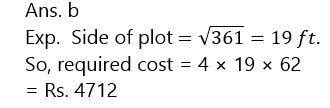# RBI Assistant Pre 2023 Quantitative Aptitude Quiz -4

## RBI Assistant Pre 2023 Quantitative Aptitude

Quantitative Aptitude is the most significant part of almost all competitive exams. Many candidates find difficulty in solving Quantitative Aptitude Questions. The only way to ace this section is to practice as many questions as possible. And, to help you practice more and more questions, we have come up with the RBI Assistant Pre 2023 Quantitative Aptitude Quiz. This RBI Assistant Pre 2023 Quantitative Aptitude Quiz is completely Free. This RBI Assistant Pre 2023 Quantitative Aptitude Quiz includes a variety of questions that are asked in exams. Candidates will be provided with a detailed solution for each question in this RBI Assistant Pre 2023 Quantitative Aptitude Quiz. This RBI Assistant Pre 2023 Quantitative Aptitude Quiz will assist candidates in better preparing for upcoming exams.

Directions (1-5): The following table shows the no. of students joined  coaching classes for four individual subjects and a class for all combined subject  in Career Power classes in Delhi region over six years. Read the question given below and answer accordingly.

No. of students joined for Career Power classes in Delhi Region for only subjectwise classes & all subject Classes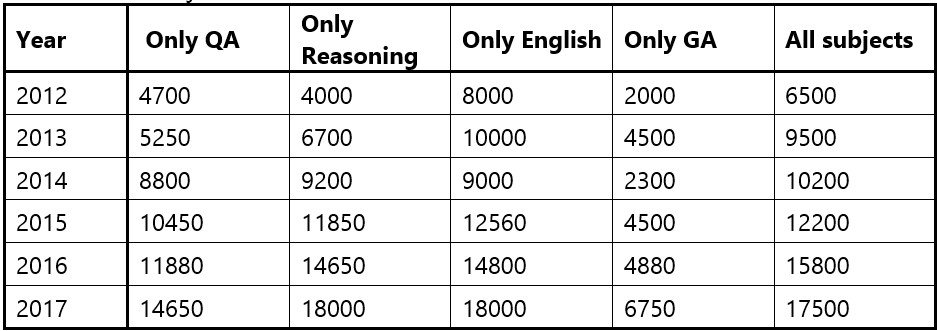1. What is total number of students who joined for QA in all the years except in 2014 ?

(a)45930

(b)46930

(c)45500

(d)12548

(e) None of these

Ans. b

Exp.  Total students = 46930

1. Number of students who joined for “All subject” classes in 2013 and 2017 together is approximately what % of  the total students who joined for Reasoning & English classes together in both  year 2013 and 2015?

(a) 66%

(b) 72.5%

(c) 70.75%

(d) 55%

(e) 69%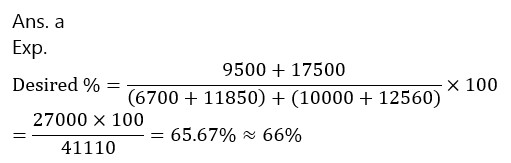1. For which of the given courses or subjects, number of students who joined is maximum over all years ?

(a)  All subjects

(b) Only QA

(c) Only GA

(d) Only Reasoning

(e) Only English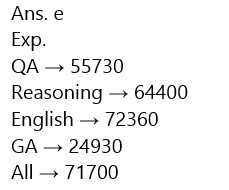1. Total students who joined for Career Power classes in 2017 is approximately what % more or less than total students who joined for G.A. subject in all the years is ?

(a) 175%

(b) 200%

(c) 180%

(d) 190%

(e) 210%1. The average of students joined for Career Power classes in QA section over all years is approximately what % of average of students joined for GA classes over all years?

(a) 210%

(b) 248%

(c) 230%

(d) 220%

(e) 200%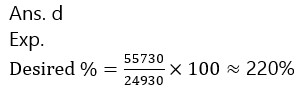1. The average of five positive numbers is 308. The average of first two numbers is 482.5 and the average of last two numbers is 258.5. What is the third number?

(a) 224

(b) 58

(c) 121

(d) Cannot be determined

(e) None of these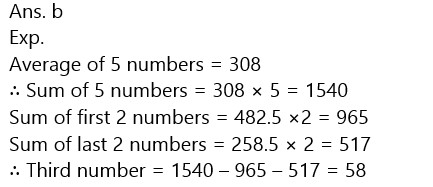1. The income of A is 150% of the income of B and the income of C is 120% of the income of A. If the total income of A, B and C together is Rs. 86,000, what is C’s income?

(a) Rs. 30,000

(b) Rs. 32,000

(c) Rs. 20,000

(d) Rs. 36,000

(e) None of these1. Alex’s expense is 30% more than Virat’s and Virat’s expense is 10% less than Ron’s. If the sum of their expenses is Rs. 6,447, then what would be Alex’s expense?

(a) Rs. 2,200

(b) Rs. 2,457

(c) Rs. 1,890

(d) Rs. 2,100

(e) None of these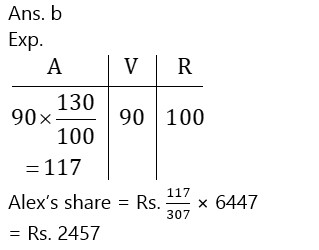1. The average marks of Science subject of a class of 20 students is 68. If the marks of two students were misread as 48 and 65 instead of the actual marks 72 and 61 respectively, what would be the correct average?

(a) 68.5

(b) 69

(c) 69.5

(d) 70

(e) 66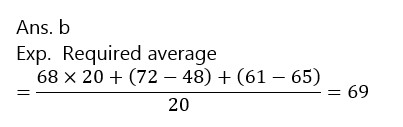1. What would be the cost of building a fence around a square plot with area equal to 361 sq. ft, if the price per foot of building the fence is Rs. 62?

(a) Rs. 4026

(b) Rs. 4712

(c) Rs. 3948

(d) Cannot be determined

(e) None of these